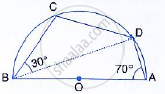Share

# In the Given Figure, C and D Are Points on the Semi-circle Described on Ab as Diameter. Given Angle Bad = 70° and Angle Dbc = 30°, Calculate Angle Bdc. - Mathematics

Course

#### Question

In the given figure, C and D are points on the semi-circle described on AB as diameter. Given angle BAD = 70° and angle DBC = 30°, calculate angle BDC.#### Solution

Since ABCD is a cyclic quadrilateral, therefore, ∠BCD + ∠BAD = 180°
(since opposite angles of a cyclic quadrilateral are supplementary)
⇒ ∠BCD + 70° = 180°
⇒ ∠BCD = 180° − 70° = 110°
In ΔBCD, we have,
∠CBD + ∠BCD + ∠BDC = 180°
⇒ 30° + 110° + ∠BDC = 180°
⇒ ∠BDC = 180° − 140°
⇒ ∠BDC = 40°

Is there an error in this question or solution?